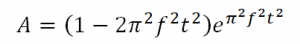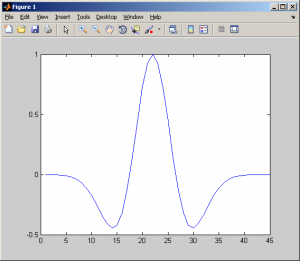# C/C++ Ricker Wavelets Code

Ricker Wavelets are zero-phase wavelets with a central peak and two smaller side lobes. A Ricker Wavelets can be uniquely specified with only a single parameter “f”. It’s peak frequency as seen on the wavelet spectrum. Ricker Wavelets Equation is :After searching in internet, I get Matlab code for this Ricker Wavelets and convert that Ricker Wavelets to C code. This is my C/C++ Ricker Wavelets Code (save code as rickerwavelets.c) :

```/*
* rickerwavelet.c
*
*  Created on: May 25, 2011
*      Author: toto
*/

#include <stdio.h>
#include <stdlib.h>
#include <math.h>
float *rickerwavelet(float fpeak, float dt, int *nwricker);
int main(int argc, char **argv)
{
int i;
float dt;
float fpeak;

float *wricker=NULL;
int nwricker;

fpeak = atof(argv);
dt = atof(argv);

wricker = rickerwavelet(fpeak, dt, &nwricker);

/* show value of ricker wavelets */
for (i=0; i<nwricker; i++)
printf("%i. %3.5f \n", i, wricker[i]);

free(wricker);
return(1);
}

/* ricker wavelet function, return an array ricker wavelets */
float *rickerwavelet(float fpeak, float dt, int *nwricker)
{
int i, k;
int nw;
int nc;
float pi;
float nw1, alpha, beta;
float *wricker=NULL;

pi = 3.141592653589793;
nw1 = 2.2/fpeak/dt;
nw = 2*floor(nw1/2)+1;
nc = floor(nw/2);

wricker = (float*) calloc (nw, sizeof(float));
for (i=0; i<nw; i++)
{
k = i+1;
alpha = (nc-k+1)*fpeak*dt*pi;
beta = pow(alpha, 2.0);
wricker[i] = (1 - (beta*2)) * exp(-beta);
}

(*nwricker) = nw;
return(wricker);
}```

Compile this C/C++ Ricker Wavelets code with command :

gcc rickerwavelets.c -lm -o rickerwavelets

Running this program with command :

rickerwavelets fpeak_value dt_value  –> example : rickerwavelets 1.0 0.05

This is sample output data from Ricker Wavelet Code and plot data in Matlab:One Comment
1.